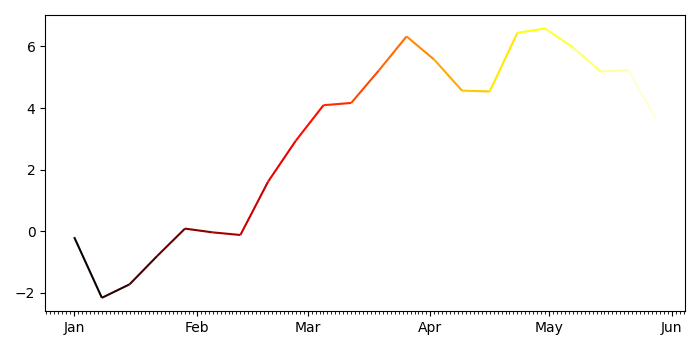# How to plot multi-color line if X-axis is datetime index of Pandas?

MatplotlibPythonData Visualization

To plot multicolor line if X-axis is datetime index of Pandas, we can take the following steps −

• Set the figure size and adjust the padding between and around the subplots.
• Create d, y and s data points using numpy.
• Create a figure and a set of subplots.
• Get xval, p and s data point using numpy.
• Get the line collection instance with hot colormap and s data points.
• Set major and minor axes locator and set axes formatter.
• Autoscale the view limits using the data limits.
• To display the figure, use show() method.

## Example

import pandas as pd
from matplotlib import pyplot as plt, dates as mdates, collections as c
import numpy as np

plt.rcParams["figure.figsize"] = [7.00, 3.50]
plt.rcParams["figure.autolayout"] = True

d = pd.date_range("2021-01-01", "2021-06-01", freq="7D")
y = np.cumsum(np.random.normal(size=len(d)))
s = pd.Series(y, index=d)

fig, ax = plt.subplots()

xval = mdates.date2num(s.index.to_pydatetime())
p = np.array([xval, s.values]).T.reshape(-1, 1, 2)
s = np.concatenate([p[:-1], p[1:]], axis=1)
lc = c.LineCollection(s, cmap="hot")
lc.set_array(xval)

ax.xaxis.set_major_locator(mdates.MonthLocator())
ax.xaxis.set_minor_locator(mdates.DayLocator())
m = mdates.DateFormatter("%b")
ax.xaxis.set_major_formatter(m)
ax.autoscale_view()

plt.show()

## Output

It will produce the following output